Last updated: 2016-11-08

Code version: bd286a36f14d3b332285cdc7e62258b1f616bb14

## Input

library("dplyr")
library("edgeR")
library("ggplot2")
library("cowplot")
theme_set(theme_bw(base_size = 16))
theme_update(panel.grid.minor.x = element_blank(),
panel.grid.minor.y = element_blank(),
panel.grid.major.x = element_blank(),
panel.grid.major.y = element_blank(),
legend.key = element_blank(),
plot.title = element_text(size = rel(1)))
source("functions.R")

Summary counts from featureCounts. Created with gather-summary-counts.py. These data were collected from the summary files of the full combined samples.

summary_per_sample <- read.table("../data/summary-counts.txt", header = TRUE,
stringsAsFactors = FALSE)
stopifnot(summary_per_sample$well != "bulk", sum(summary_per_sample$rmdup == "reads") == 864,
sum(summary_per_sample$rmdup == "molecules") == 864) Remove featureCounts classifications with zero counts. stopifnot(colSums(summary_per_sample[, c(7, 10:15)]) == 0) summary_per_sample <- summary_per_sample[, c(-7, -10:-15)] head(summary_per_sample)  individual replicate well rmdup Assigned Unassigned_Ambiguity 1 NA19098 r1 A01 molecules 63322 1419 2 NA19098 r1 A01 reads 1932782 40278 3 NA19098 r1 A02 molecules 63976 1454 4 NA19098 r1 A02 reads 2039613 44664 5 NA19098 r1 A03 molecules 43630 976 6 NA19098 r1 A03 reads 1006487 18865 Unassigned_NoFeatures Unassigned_Unmapped 1 54297 0 2 885075 1093943 3 49595 0 4 737166 1140902 5 33597 0 6 419226 742614 Input annotation. anno <- read.table("../data/annotation.txt", header = TRUE, stringsAsFactors = FALSE) stopifnot(anno$well != "bulk", nrow(anno) == 864,
rep(anno$individual, each = 2) == summary_per_sample$individual,
rep(anno$replicate, each = 2) == summary_per_sample$replicate,
rep(anno$well, each = 2) == summary_per_sample$well)
head(anno)
  individual replicate well      batch      sample_id
1    NA19098        r1  A01 NA19098.r1 NA19098.r1.A01
2    NA19098        r1  A02 NA19098.r1 NA19098.r1.A02
3    NA19098        r1  A03 NA19098.r1 NA19098.r1.A03
4    NA19098        r1  A04 NA19098.r1 NA19098.r1.A04
5    NA19098        r1  A05 NA19098.r1 NA19098.r1.A05
6    NA19098        r1  A06 NA19098.r1 NA19098.r1.A06

reads <- read.table("../data/reads.txt", header = TRUE,
stringsAsFactors = FALSE)
colnames(reads) == anno$sample_id) Input molecule counts. molecules <- read.table("../data/molecules.txt", header = TRUE, stringsAsFactors = FALSE) stopifnot(ncol(molecules) == nrow(anno), colnames(reads) == anno$sample_id)

Input single cell observational quality control data.

qc <- read.table("../data/qc-ipsc.txt", header = TRUE,
stringsAsFactors = FALSE)
stopifnot(qc$individual == anno$individual,
qc$replicate == anno$replicate,
qc$well == anno$well)
head(qc)
  individual replicate well cell_number concentration tra1.60
1    NA19098        r1  A01           1      1.734785       1
2    NA19098        r1  A02           1      1.723038       1
3    NA19098        r1  A03           1      1.512786       1
4    NA19098        r1  A04           1      1.347492       1
5    NA19098        r1  A05           1      2.313047       1
6    NA19098        r1  A06           1      2.056803       1

## Total ERCC and removal of NA19098.r2

Show the evidence that removing NA19098 batch 2 is the first thing to do.

summary_per_sample_reads <- summary_per_sample[summary_per_sample$rmdup == "reads",] summary_per_sample_reads$sample_id <- anno$sample_id summary_per_sample_reads$batch <- anno$batch stopifnot(colnames(reads) == summary_per_sample_reads$sample_id )

summary_per_sample_reads$ERCC_reads <- apply(reads[grep("ERCC", rownames(reads)), ],2,sum) summary_per_sample_reads$ERCC_molecules <- apply(molecules[grep("ERCC", rownames(molecules)), ],2,sum)
## create a color palette with one color per individual and different shades for repplicates
great_color <- c("#CC3300", "#FF9966", "#FFCC99", "#006633", "#009900", "#99FF99", "#3366FF", "#6699FF", "#66CCFF")
great_color_8 <- c("#CC3300", "#FF9966", "#006633", "#009900", "#99FF99", "#3366FF", "#6699FF", "#66CCFF")

aes(x = factor(batch), y = ERCC_reads,
fill = factor(batch)), height = 600, width = 2000) +
geom_violin(alpha = .5) +
geom_boxplot(alpha = .01, width = .2, position = position_dodge(width = .9)) +
scale_fill_manual(values = great_color) +
labs(x = "", y = "Total ERCC read-counts per cell",
title = "Excess amount of ERCC in NA19098.r2") +
theme(axis.text.x = element_text(hjust=1, angle = 45))

aes(x = factor(batch), y = ERCC_molecules,
fill = factor(batch)), height = 600, width = 2000) +
geom_violin(alpha = .5) +
geom_boxplot(alpha = .01, width = .2, position = position_dodge(width = .9)) +
scale_fill_manual(values = great_color) +
labs(x = "", y = "Total ERCC molecule-counts per cell",
title = "Excess amount of ERCC in NA19098.r2") +
theme(axis.text.x = element_text(hjust=1, angle = 45))

ercc_molecule_plot + theme(legend.position = "none"),
labels = LETTERS[1:2])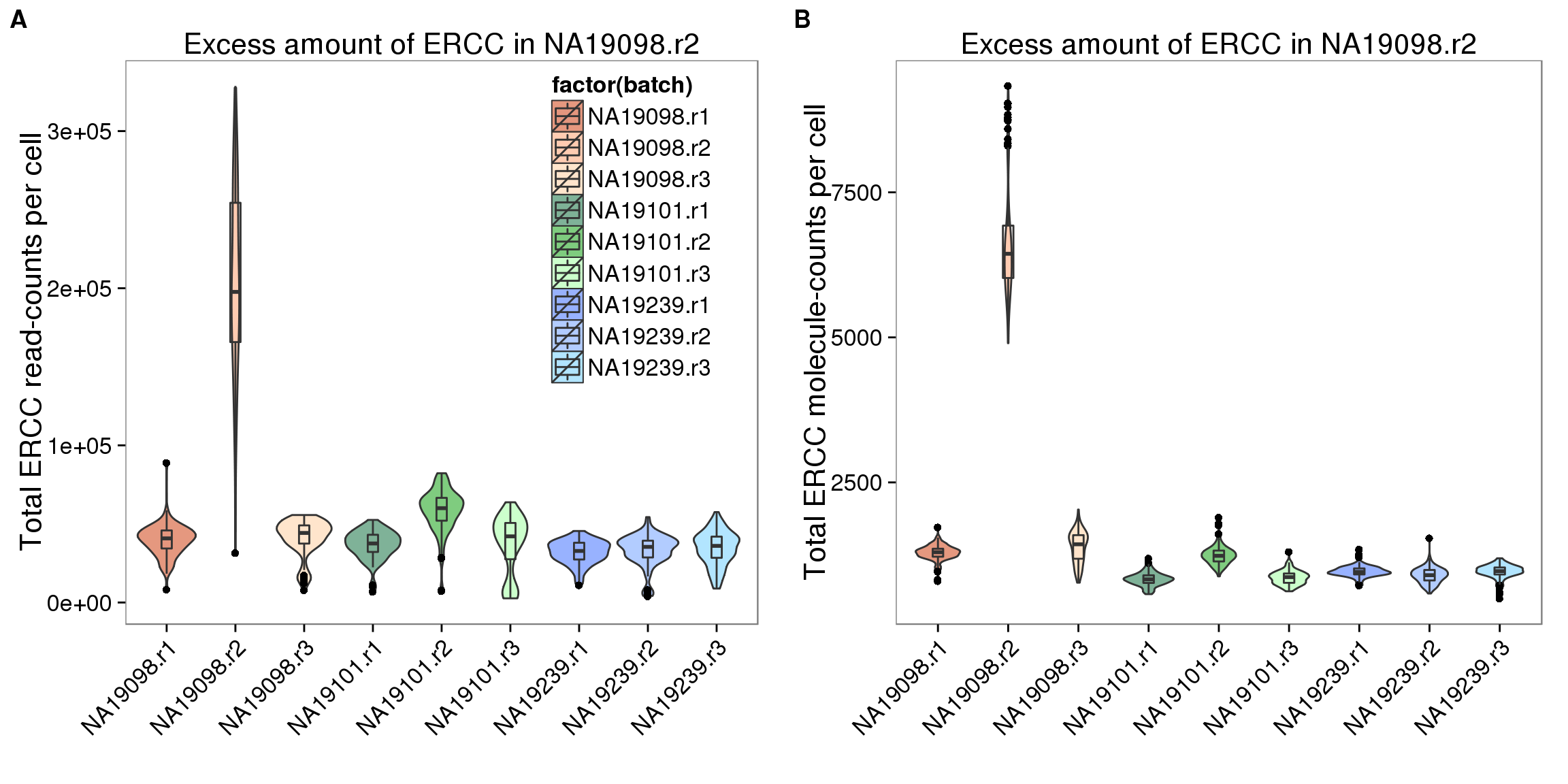Remove NA19098r2 for all the following analysis

remove_19098r2 <- anno$batch != "NA19098.r2" anno_rm <- anno[remove_19098r2,] summary_per_sample_reads_rm <- summary_per_sample_reads[remove_19098r2,] reads_rm <- reads[, remove_19098r2] molecules_rm <- molecules[, remove_19098r2] stopifnot(summary_per_sample_reads_rm$sample_id == colnames(reads_rm))

## add cell number per well by merging qc file

## calculate total mapped reads per sample
summary_per_sample_reads_qc$total_mapped <- apply(summary_per_sample_reads_qc[,5:7],1,sum) ## cut off cut_off_reads <- quantile(summary_per_sample_reads_qc[summary_per_sample_reads_qc$cell_number == 0,"total_mapped"], 0.95)

cut_off_reads
    95%
1556255 
summary_per_sample_reads_qc$cut_off_reads <- summary_per_sample_reads_qc$total_mapped > cut_off_reads

## numbers of cells
sum(summary_per_sample_reads_qc[summary_per_sample_reads_qc$cell_number == 1, "total_mapped"] > cut_off_reads)  603 sum(summary_per_sample_reads_qc[summary_per_sample_reads_qc$cell_number == 1, "total_mapped"] <= cut_off_reads)
 96
## density plots
plot_reads <- ggplot(summary_per_sample_reads_qc[summary_per_sample_reads_qc$cell_number == 0 | summary_per_sample_reads_qc$cell_number == 1 , ],
aes(x = total_mapped, fill = as.factor(cell_number))) +
geom_density(alpha = 0.5) +
geom_vline(xintercept = cut_off_reads, colour="grey", linetype = "longdash") +
labs(x = "Total mapped reads per sample", title = "Cutoff based on the number of total mapped reads", fill = "Cell number")

## Unmapped ratios

## calculate unmapped ratios
summary_per_sample_reads_qc$unmapped_ratios <- summary_per_sample_reads_qc[,8]/apply(summary_per_sample_reads_qc[,5:8],1,sum) ## cut off cut_off_unmapped <- quantile(summary_per_sample_reads_qc[summary_per_sample_reads_qc$cell_number == 0,"unmapped_ratios"], 0.05)

cut_off_unmapped
       5%
0.3640165 
summary_per_sample_reads_qc$cut_off_unmapped <- summary_per_sample_reads_qc$unmapped_ratios < cut_off_unmapped

## numbers of cells
sum(summary_per_sample_reads_qc[summary_per_sample_reads_qc$cell_number == 1, "unmapped_ratios"] >= cut_off_unmapped)  101 sum(summary_per_sample_reads_qc[summary_per_sample_reads_qc$cell_number == 1, "unmapped_ratios"] < cut_off_unmapped)
 598
## density plots
plot_unmapped <- ggplot(summary_per_sample_reads_qc[summary_per_sample_reads_qc$cell_number == 0 | summary_per_sample_reads_qc$cell_number == 1 , ],
aes(x = unmapped_ratios *100, fill = as.factor(cell_number))) +
geom_density(alpha = 0.5) +
geom_vline(xintercept = cut_off_unmapped *100, colour="grey", linetype = "longdash") +
labs(x = "Unmapped reads / Total reads per sample", title = "Cutoff based on the percentage unmapped reads")

## ERCC percentage

## calculate ercc reads percentage
summary_per_sample_reads_qc$ercc_percentage <- apply(reads_rm[grep("ERCC", rownames(reads_rm)), ],2,sum)/apply(summary_per_sample_reads_qc[,5:7],1,sum) ## cut off cut_off_ercc <- quantile(summary_per_sample_reads_qc[summary_per_sample_reads_qc$cell_number == 0,"ercc_percentage"], 0.05)

cut_off_ercc
       5%
0.0323008 
summary_per_sample_reads_qc$cut_off_ercc <- summary_per_sample_reads_qc$ercc_percentage < cut_off_ercc

## numbers of cells
sum(summary_per_sample_reads_qc[summary_per_sample_reads_qc$cell_number == 1, "ercc_percentage"] >= cut_off_ercc)  90 sum(summary_per_sample_reads_qc[summary_per_sample_reads_qc$cell_number == 1, "ercc_percentage"] < cut_off_ercc)
 609
## density plots
plot_ercc <- ggplot(summary_per_sample_reads_qc[summary_per_sample_reads_qc$cell_number == 0 | summary_per_sample_reads_qc$cell_number == 1 , ],
aes(x = ercc_percentage *100, fill = as.factor(cell_number))) +
geom_density(alpha = 0.5) +
geom_vline(xintercept = cut_off_ercc *100, colour="grey", linetype = "longdash") +
labs(x = "ERCC reads / Total mapped reads per sample", title = "Cutoff based on the percentage of ERCC reads")

## Number of genes detected

## endogenous genes

## number of genes detected
summary_per_sample_reads_qc$gene_number <- colSums(reads_rm_gene >= 1) ## cut off cut_off_genes <- quantile(summary_per_sample_reads_qc[summary_per_sample_reads_qc$cell_number == 0,"gene_number"], 0.95)

cut_off_genes
   95%
6788.9 
summary_per_sample_reads_qc$cut_off_genes <- summary_per_sample_reads_qc$gene_number > cut_off_genes

## numbers of cells
sum(summary_per_sample_reads_qc[summary_per_sample_reads_qc$cell_number == 1, "gene_number"] > cut_off_genes)  629 sum(summary_per_sample_reads_qc[summary_per_sample_reads_qc$cell_number == 1, "gene_number"] <= cut_off_genes)
 70
## density plots
plot_gene <- ggplot(summary_per_sample_reads_qc[summary_per_sample_reads_qc$cell_number == 0 | summary_per_sample_reads_qc$cell_number == 1 , ],
aes(x = gene_number, fill = as.factor(cell_number))) +
geom_density(alpha = 0.5) +
geom_vline(xintercept = cut_off_genes, colour="grey", linetype = "longdash") +
labs(x = "Gene numbers per sample", title = "Cutoff based on the number of detected genes")
plot_grid(plot_reads + theme(legend.position=c(.7,.7)),
plot_unmapped + theme(legend.position = "none"),
plot_ercc + theme(legend.position = "none"),
plot_gene + theme(legend.position = "none"),
labels = LETTERS[3:6])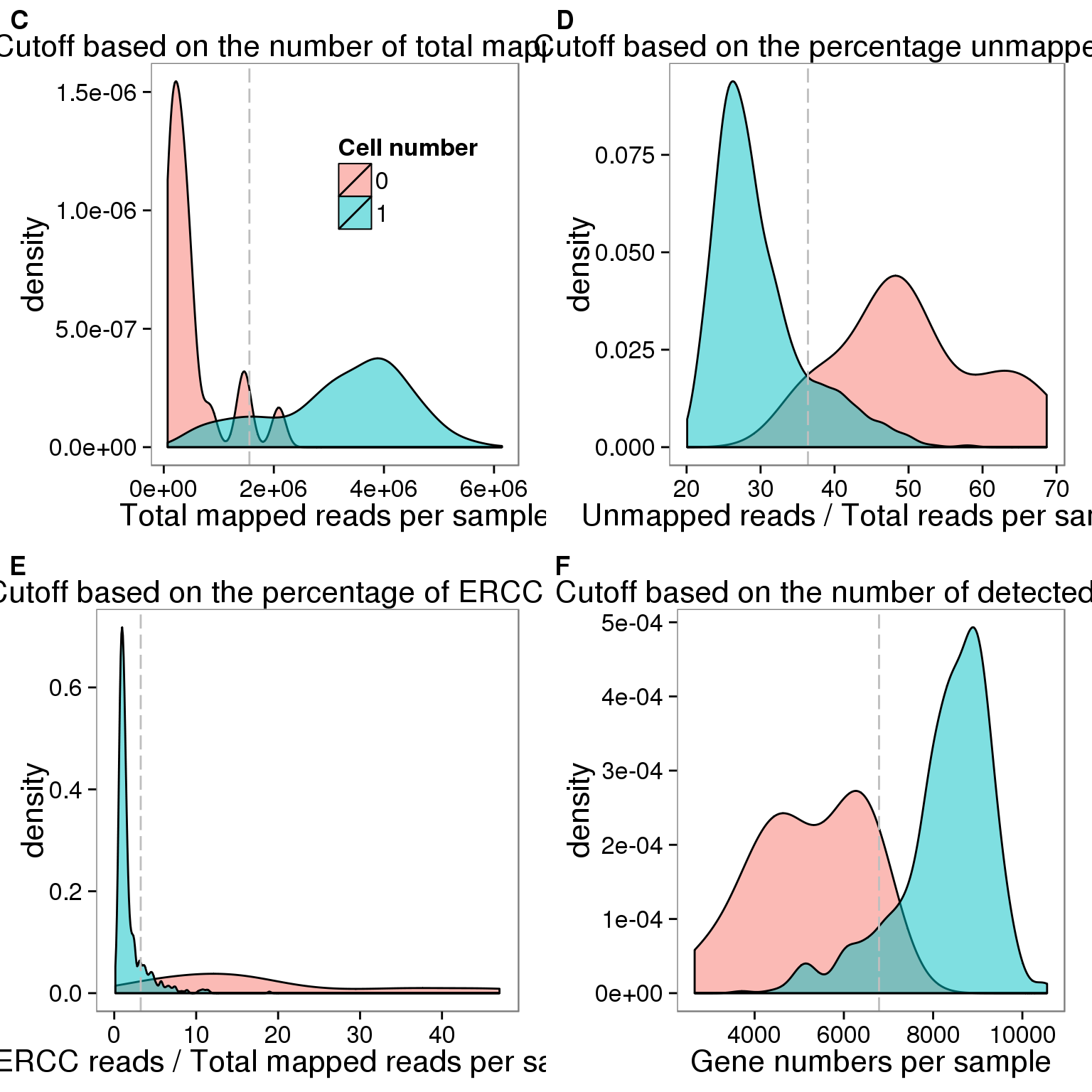## Total molecule counts

## calculate total gene molecule counts
summary_per_sample_reads_qc$total_gene_molecule <- colSums(molecules_rm[grep("ENSG", rownames(molecules_rm)),]) ## look for outiers ggplot(summary_per_sample_reads_qc, aes(x = concentration, y = total_gene_molecule / 10^3, color = as.factor(cell_number))) + geom_text(aes(label = cell_number)) + labs(x = "Concentration", y = "Gene molecules (thousands)") + scale_color_brewer(palette = "Dark2") + theme(legend.position = "none")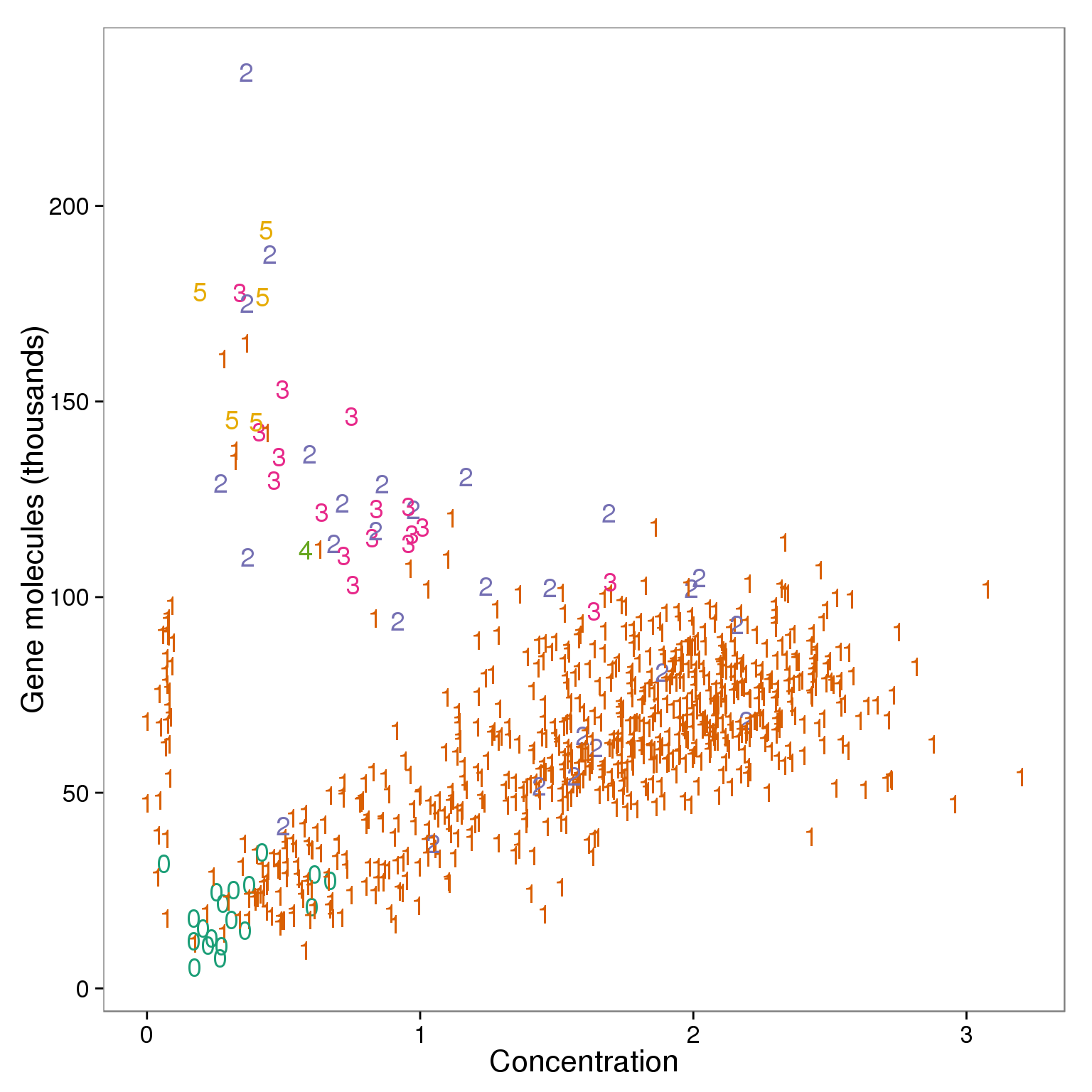outliers <- summary_per_sample_reads_qc %>% filter(cell_number == 1, concentration < 1.25, concentration > .15, total_gene_molecule > 100000) outliers %>% dplyr::select(sample_id)  sample_id 1 NA19098.r3.B04 2 NA19098.r3.B11 3 NA19101.r1.B10 4 NA19101.r2.D07 5 NA19101.r3.C07 6 NA19101.r3.D08 7 NA19101.r3.F05 8 NA19101.r3.F10 9 NA19239.r2.A12 10 NA19239.r2.B07 ### Linear Discriminant Analysis library(MASS)  Attaching package: 'MASS' The following object is masked from 'package:dplyr': select ## create 3 groups according to cell number group_3 <- rep("multiple cells",dim(summary_per_sample_reads_qc)) group_3[grep("0", summary_per_sample_reads_qc$cell_number)] <- "no cells"
group_3[grep("1", summary_per_sample_reads_qc$cell_number)] <- "one cell" ## create data frame data_lda <- data.frame(anno_rm, cell_number = summary_per_sample_reads_qc$cell_number,
concentration = summary_per_sample_reads_qc$concentration, total_gene_molecule = summary_per_sample_reads_qc$total_gene_molecule,
group = group_3)

## remove 19098.r1
data_con <- data_lda %>% filter(batch != "NA19098.r1")
plot_before <- ggplot(data_con, aes(x = concentration, y = total_gene_molecule / 10^3,
color = as.factor(group))) +
geom_text(aes(label = cell_number)) +
labs(x = "Sample concentration",
y = "Total gene molecule-count per sample (thousands)",
title = "Before linear discriminant analysis (LDA) \n A few samples with one cell (purple) \n clustered with samples with multiple cells (green)") +
scale_color_brewer(palette = "Dark2") +
theme(legend.position = "none")

## perform lda
data_con_lda <- lda(group ~ concentration + total_gene_molecule,
data = data_con)
data_con_lda_p <- predict(data_con_lda,
newdata = data_con[,c("concentration", "total_gene_molecule")])$class ## determine how well the model fix table(data_con_lda_p, data_con[, "group"])  data_con_lda_p multiple cells no cells one cell multiple cells 34 0 10 no cells 0 1 0 one cell 15 16 596 data_con$data_con_lda_p <- data_con_lda_p

plot_after <- ggplot(data_con, aes(x = concentration, y = total_gene_molecule / 10^3,
color = as.factor(data_con_lda_p))) +
geom_text(aes(label = cell_number)) +
labs(x = "Sample concentration",
y = "Total gene molecule-count per sample (thousands)",
title = "After linear discriminant analysis (LDA) \n Removal of samples classified as \n samples with multiple cells (green)") +
scale_color_brewer(palette = "Dark2") +
theme(legend.position = "none")

## identify the outlier
outliers_lda <- data_con %>% filter(cell_number == 1, data_con_lda_p == "multiple cells")
outliers_lda$sample_id   "NA19098.r3.B04" "NA19098.r3.B11" "NA19101.r1.B10" "NA19101.r2.D07"  "NA19101.r3.C07" "NA19101.r3.D08" "NA19101.r3.F05" "NA19101.r3.F10"  "NA19239.r2.A12" "NA19239.r2.B07" ## The lds method identifies outliers plot_grid(plot_before + theme(legend.position=c(.8,.85)), plot_after + theme(legend.position = "none"), labels = LETTERS[1:2])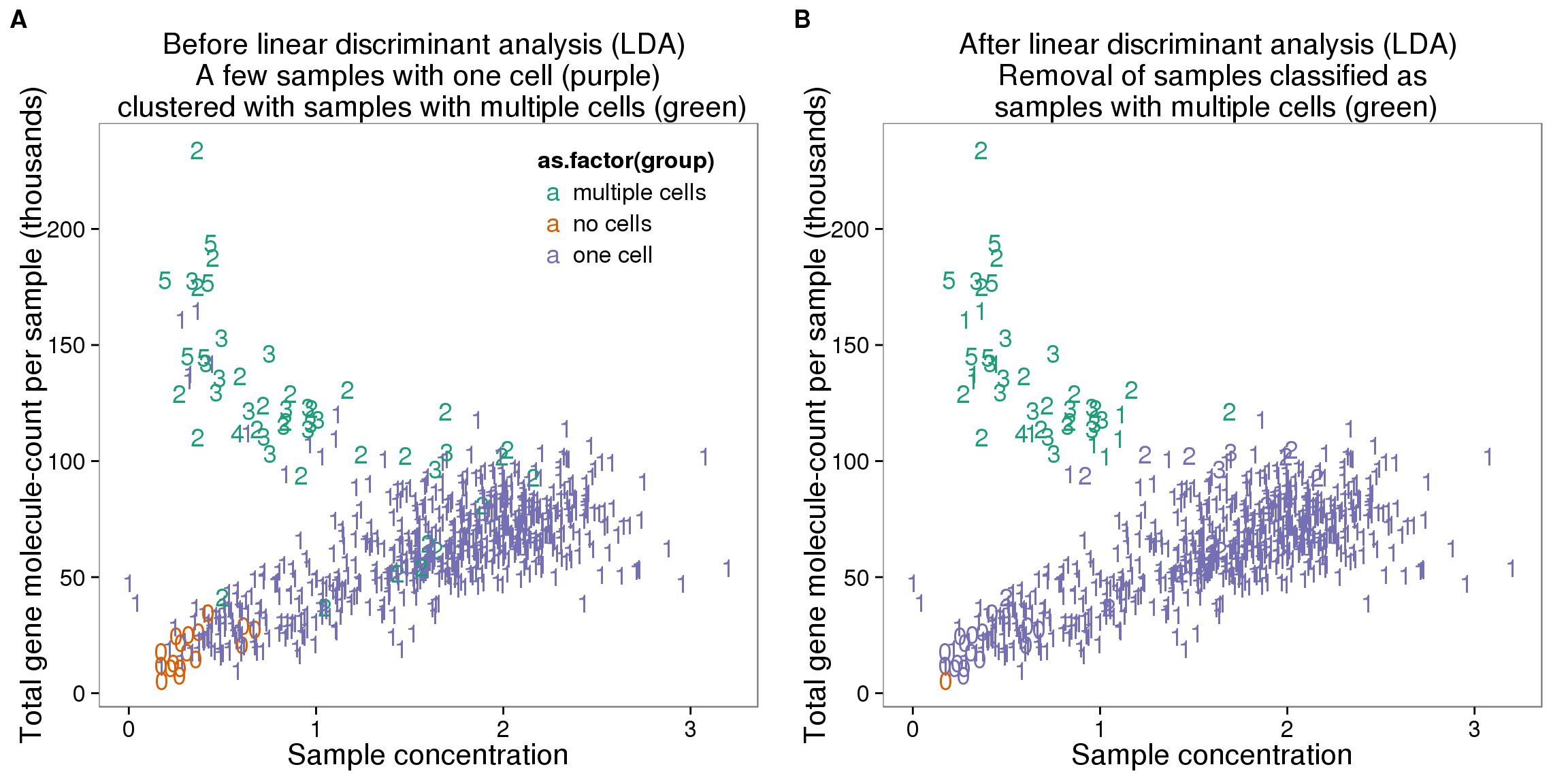## create filter summary_per_sample_reads_qc$molecule_outlier <- summary_per_sample_reads_qc$sample_id %in% outliers_lda$sample_id

## calculate convertion
summary_per_sample_reads_qc$ERCC_conversion <- summary_per_sample_reads_qc$ERCC_molecules / summary_per_sample_reads_qc$ERCC_reads summary_per_sample_reads_qc$conversion <- summary_per_sample_reads_qc$total_gene_molecule / colSums(reads_rm[grep("ENSG", rownames(reads_rm)),]) ggplot(summary_per_sample_reads_qc, aes(x = ERCC_conversion, y = conversion, color = as.factor(cell_number))) + geom_text(aes(label = cell_number)) + labs(x = "Convertion of ERCC", y = "Conversion of genes") + scale_color_brewer(palette = "Dark2") + theme(legend.position = "none")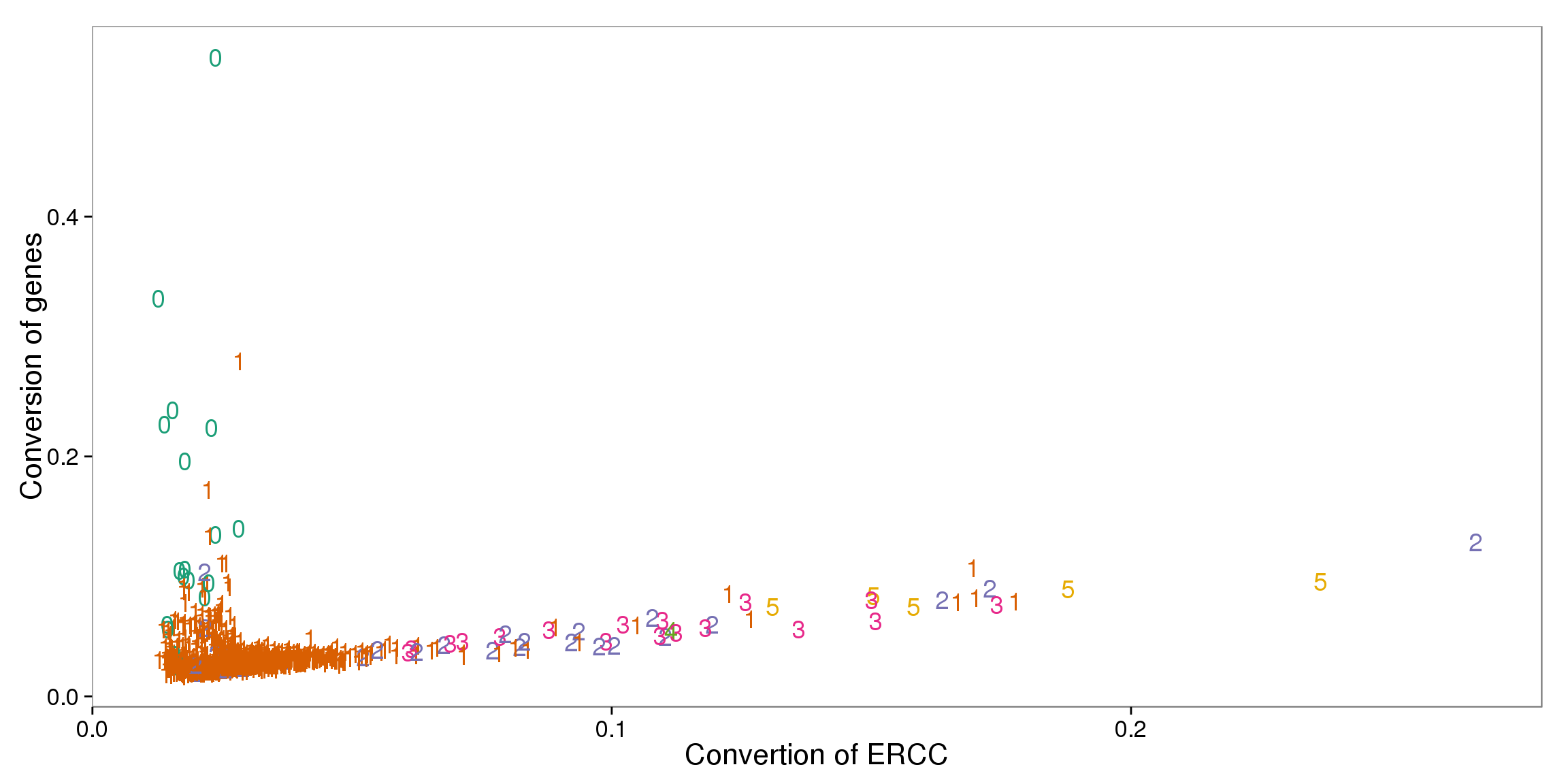out_ercc_con <- summary_per_sample_reads_qc %>% filter(cell_number == "1", ERCC_conversion > .094) ## try lda data_lda$conversion <- summary_per_sample_reads_qc$conversion data_lda$ERCC_conversion <- summary_per_sample_reads_qc$ERCC_conversion data_ercc_lda <- lda(group ~ ERCC_conversion + conversion, data = data_lda) data_ercc_lda_p <- predict(data_ercc_lda, newdata = data_lda[,c("ERCC_conversion", "conversion")])$class
table(data_con_lda_p, data_con[, "group"])

data_con_lda_p   multiple cells no cells one cell
multiple cells             34        0       10
no cells                    0        1        0
one cell                   15       16      596
data_lda$data_ercc_lda_p <- data_ercc_lda_p ## identify the outlier outliers_ercc <- data_lda %>% filter(cell_number == 1, data_ercc_lda_p == "multiple cells") outliers_ercc$sample_id
  "NA19098.r1.F01" "NA19098.r3.B04" "NA19098.r3.B11" "NA19101.r2.D07"
 "NA19101.r3.C07" "NA19101.r3.D08" "NA19101.r3.F05" "NA19101.r3.F10"
 "NA19239.r2.A12" "NA19239.r2.B07" "NA19239.r3.G02"
## cutoff
out_ercc_con <- summary_per_sample_reads_qc %>% filter(cell_number == "1", ERCC_conversion > .08)

## create filter
summary_per_sample_reads_qc$conversion_outlier <- summary_per_sample_reads_qc$sample_id %in% outliers_ercc$sample_id plot_ercc_before <- ggplot(data_lda, aes(x = ERCC_conversion, y = conversion, color = as.factor(group))) + geom_text(aes(label = cell_number)) + labs(x = "Read-to-molecule conversion of ERCC", y = "Read-to-molecule conversion of genes", title = "Before linear discriminant analysis (LDA) \n A few samples with one cell (purple) \n clustered with samples with multiple cells (green)") + scale_color_brewer(palette = "Dark2") + theme(legend.position = "none") plot_ercc_after <- ggplot(data_lda, aes(x = ERCC_conversion, y = conversion, color = as.factor(data_ercc_lda_p))) + geom_text(aes(label = cell_number)) + labs(x = "Read-to-molecule conversion of ERCC", y = "Read-to-molecule conversion of genes", title = "After linear discriminant analysis (LDA) \n Removal of samples classified as \n samples with multiple cells (green)") + scale_color_brewer(palette = "Dark2") + theme(legend.position = "none") plot_grid(plot_ercc_before, plot_ercc_after, labels = LETTERS[3:4])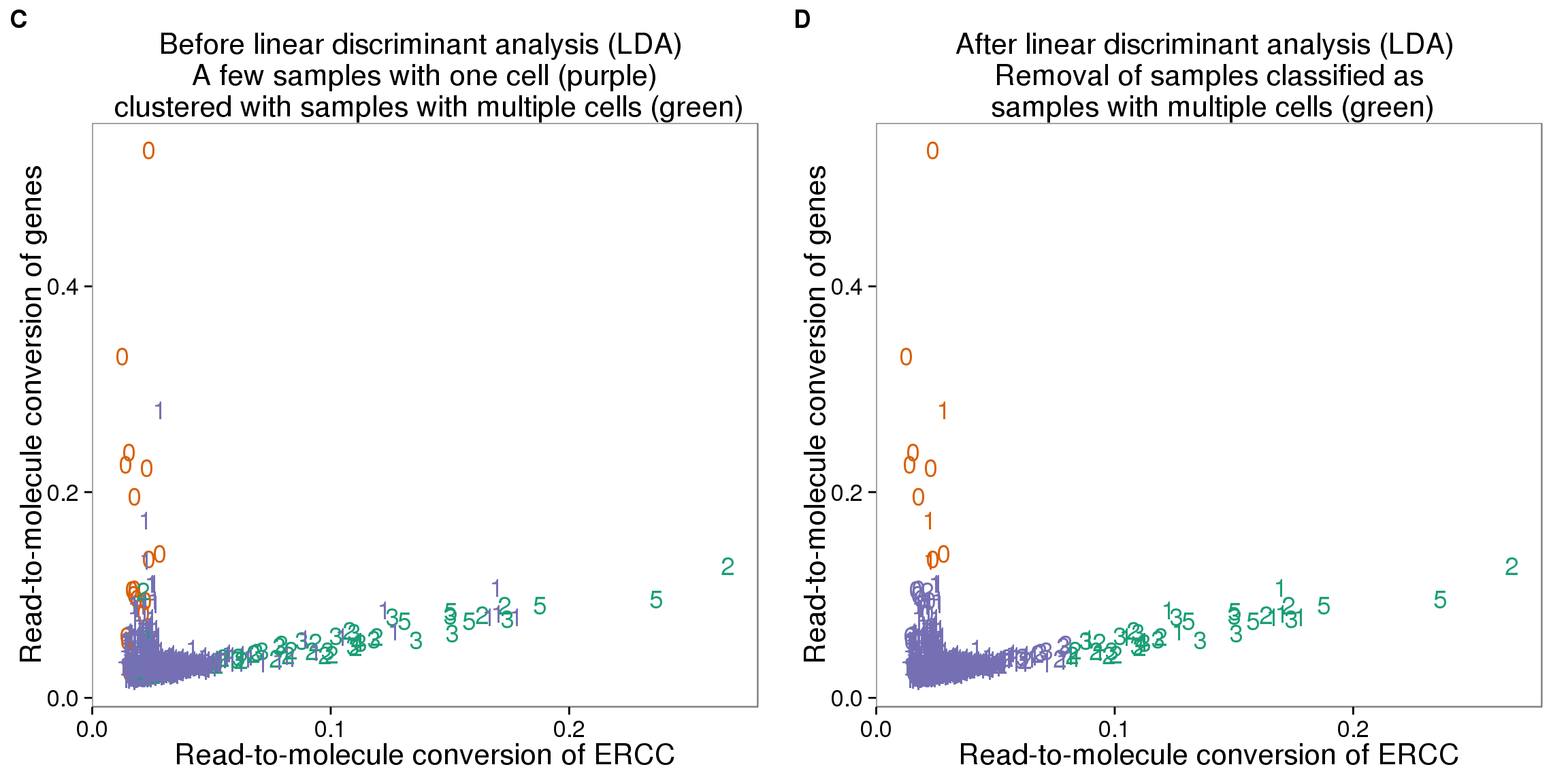## Mitochondrial genes ## create a list of mitochondrial genes (13 protein-coding genes) ## MT-ATP6, MT-CYB, MT-ND1, MT-ND4, MT-ND4L, MT-ND5, MT-ND6, MT-CO2, MT-CO1, MT-ND2, MT-ATP8, MT-CO3, MT-ND3 mtgene <- c("ENSG00000198899", "ENSG00000198727", "ENSG00000198888", "ENSG00000198886", "ENSG00000212907", "ENSG00000198786", "ENSG00000198695", "ENSG00000198712", "ENSG00000198804", "ENSG00000198763","ENSG00000228253", "ENSG00000198938", "ENSG00000198840") ## reads of mt genes in single cells mt_reads <- reads_rm_gene[mtgene,] dim(mt_reads)  13 768 stopifnot(colnames(reads_rm) == rownames(summary_per_sample_reads_qc$sample_id))

## mt ratio of single cell
summary_per_sample_reads_qc$mt_reads <- apply(mt_reads, 2, sum) summary_per_sample_reads_qc$mt_reads_ratio <- summary_per_sample_reads_qc$mt_reads /summary_per_sample_reads_qc$total_mapped

## vs. number of genes detected
aes(x = gene_number, y = mt_reads_ratio,
color = as.factor(cell_number))) +
geom_text(aes(label = cell_number)) +
labs(x = "Number of genes", y = "Mitochondrial ratio") +
scale_color_brewer(palette = "Dark2") +
theme(legend.position = "none")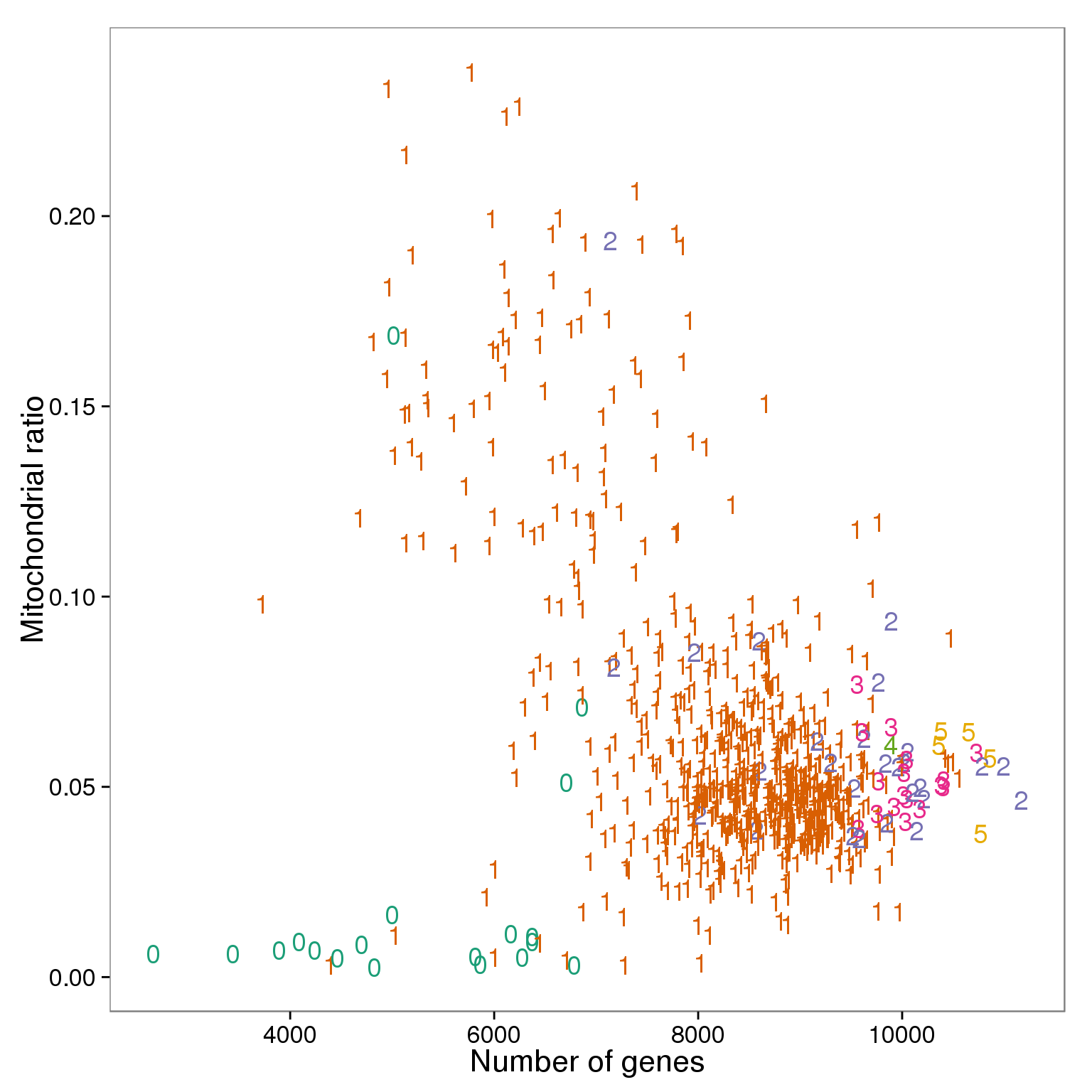## Filter

### Final list

## all filter
summary_per_sample_reads_qc$filter_all <- summary_per_sample_reads_qc$cell_number == 1 &
summary_per_sample_reads_qc$cut_off_reads & summary_per_sample_reads_qc$cut_off_unmapped &
summary_per_sample_reads_qc$cut_off_ercc & summary_per_sample_reads_qc$cut_off_genes &
summary_per_sample_reads_qc$molecule_outlier == "FALSE" & summary_per_sample_reads_qc$conversion_outlier == "FALSE"

table(summary_per_sample_reads_qc[summary_per_sample_reads_qc$filter_all, c("individual", "replicate")])   replicate individual r1 r2 r3 NA19098 85 0 57 NA19101 80 70 51 NA19239 74 68 79 stopifnot(nrow(summary_per_sample_reads_qc) == nrow(anno_rm)) quality_single_cells <- anno_rm %>% filter(summary_per_sample_reads_qc$filter_all) %>%
dplyr :: select(sample_id)

write.table(quality_single_cells,
file = "../data/quality-single-cells.txt", quote = FALSE,
sep = "\t", row.names = FALSE, col.names = FALSE)

### Mito ratios

ggplot(summary_per_sample_reads_qc,
aes(x = gene_number, y = mt_reads_ratio,
color = as.factor(filter_all))) +
geom_text(aes(label = cell_number)) +
labs(x = "Number of genes", y = "Mitochondrial ratio") +
theme(legend.position = "none")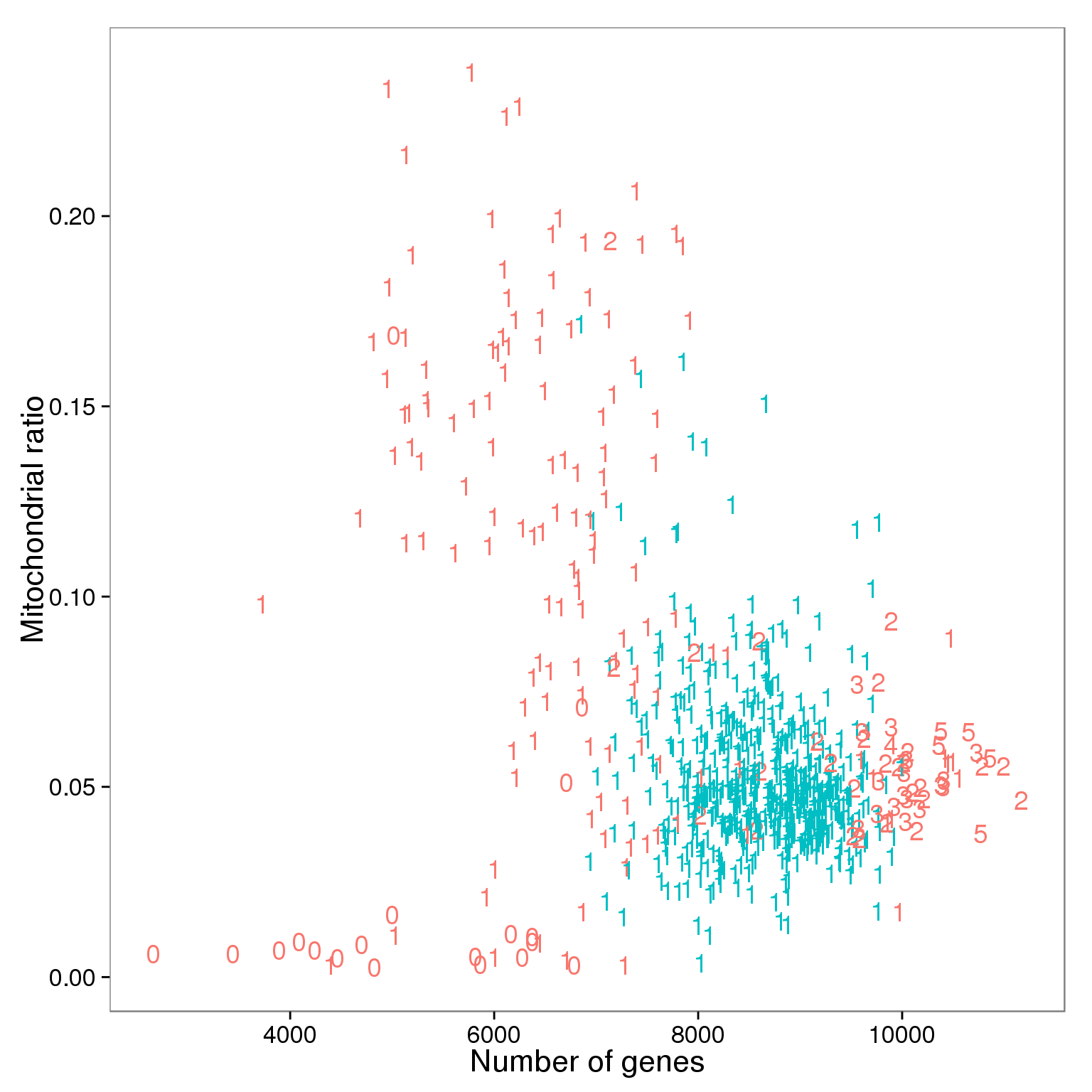ggplot(summary_per_sample_reads_qc[summary_per_sample_reads_qc$cell_number == 1,], aes(x = factor(filter_all), y = mt_reads_ratio, fill = factor(filter_all)), height = 600, width = 2000) + geom_violin(alpha = .5) + geom_boxplot(alpha = .01, width = .2, position = position_dodge(width = .9)) + labs(x = "Quality", y = "Mitochonrial ratio", title = "Mitochondrial ratio of libraries with 1 cell")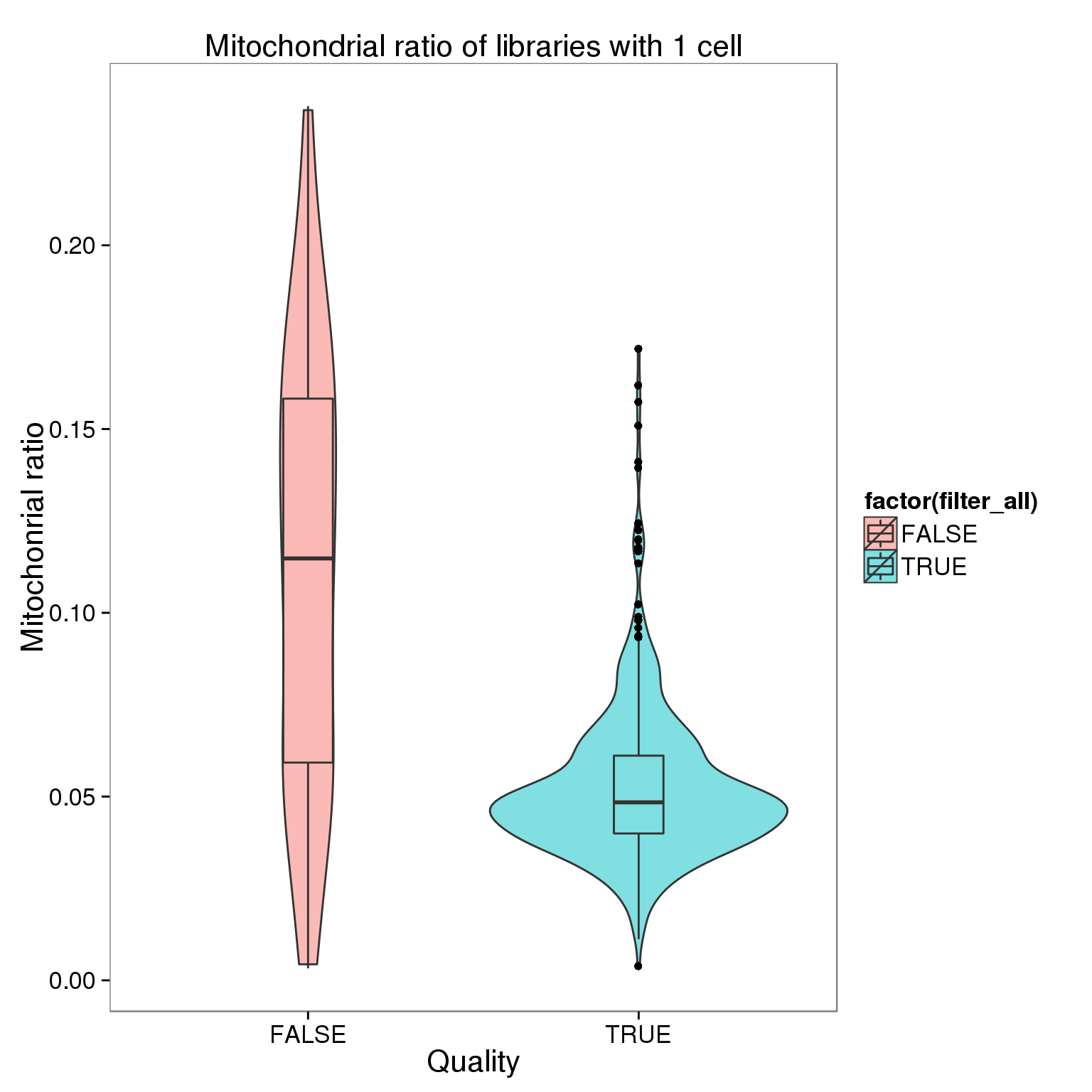## check the batch of those outliers mito_outliers <- summary_per_sample_reads_qc %>% filter(filter_all == "TRUE", mt_reads_ratio > .15) mito_outliers %>% dplyr::select(sample_id, mt_reads_ratio)  sample_id mt_reads_ratio 1 NA19098.r1.D07 0.1573689 2 NA19098.r3.G03 0.1618823 3 NA19098.r3.G04 0.1508871 4 NA19101.r3.A09 0.1718202 ## check if 19098 have high mt genes ggplot(summary_per_sample_reads_qc[summary_per_sample_reads_qc$filter_all == "TRUE",],
aes(x = factor(batch), y = mt_reads_ratio,
fill = factor(batch)), height = 600, width = 2000) +
geom_violin(alpha = .5) +
geom_boxplot(alpha = .01, width = .2, position = position_dodge(width = .9)) +
scale_fill_manual(values = great_color_8) +
labs(x = "batch", y = "Mitochondrial ratio") +
theme(axis.text.x = element_text(hjust=1, angle = 45))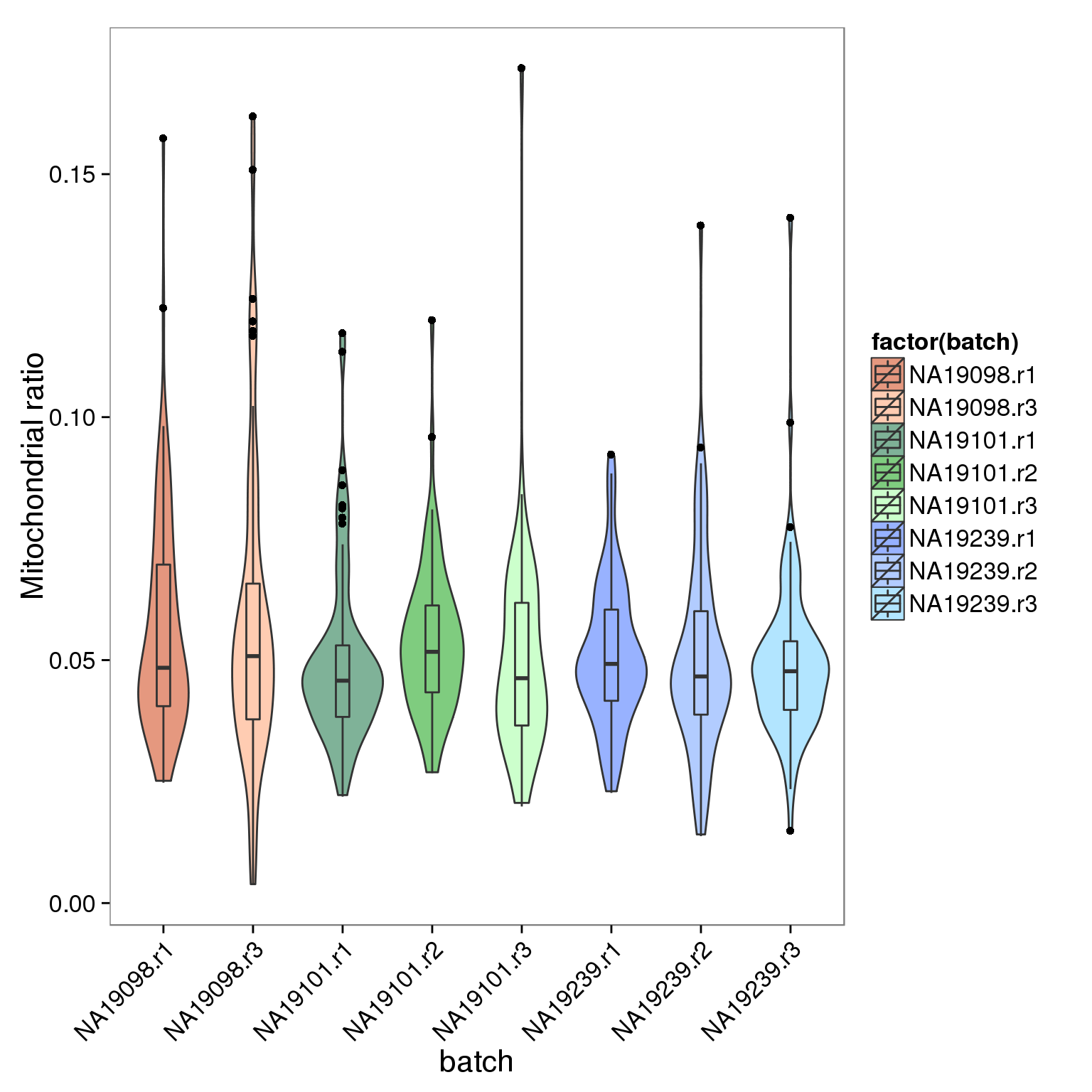### plots

genes_unmapped <-  ggplot(summary_per_sample_reads_qc,
aes(x = gene_number, y = unmapped_ratios * 100,
col = as.factor(individual), height = 600, width = 2000)) +
geom_point(size = 3, alpha = 0.3) +
geom_vline(xintercept = cut_off_genes, colour="grey", linetype = "longdash") +
geom_hline(yintercept = cut_off_unmapped * 100, colour="grey", linetype = "longdash") +
labs(x = "Number of detected genes / sample",
y = "Percentage of unmapped reads in each sample (%)",
title = "Number of genes detected as \n expressed as a function of \n the proportion of mapped reads")

aes(x = gene_number, y = ercc_percentage * 100,
col = as.factor(individual), shape = as.factor(replicate), height = 600, width = 2000)) +
geom_point(size = 3, alpha = 0.3) +
geom_vline(xintercept = cut_off_genes, colour="grey", linetype = "longdash") +
geom_hline(yintercept = cut_off_ercc * 100, colour="grey", linetype = "longdash") +
labs(x = "Number of detected genes / samlpe",
y = "Percentage of ERCC reads in each sample (%)",
title = "Samples with zero cell \n (visual inspection) have \n low number of reads")

aes(x = total_mapped, y = unmapped_ratios * 100,
col = as.factor(individual), label = as.character(cell_number), height = 600, width = 2000)) +
geom_text(fontface = 3, alpha = 0.5) +
geom_vline(xintercept = cut_off_reads, colour="grey", linetype = "longdash") +
geom_hline(yintercept = cut_off_unmapped * 100, colour="grey", linetype = "longdash") +
labs(x = "Total mapped reads / sample",
y = "Percentage of unmapped reads in each sample (%)",
title = "Samples with zero cells \n (visual inspection) have \n high proportion of unmapped reads")

aes(x = total_mapped, y = ercc_percentage * 100,
col = as.factor(individual), label = as.character(cell_number), height = 600, width = 2000)) +
geom_text(fontface = 3, alpha = 0.5) +
geom_vline(xintercept = cut_off_reads, colour="grey", linetype = "longdash") +
geom_hline(yintercept = cut_off_ercc * 100, colour="grey", linetype = "longdash") +
labs(x = "Total mapped reads / sample",
y = "Percentage of ERCC reads in each sample (%)",
title = "Samples with zero cells \n (visual inspection) have \n high proportion of ERCC reads")

plot_grid(genes_unmapped + theme(legend.position = "none"),
genes_ercc + theme(legend.position = "none"),
labels = letters[1:4])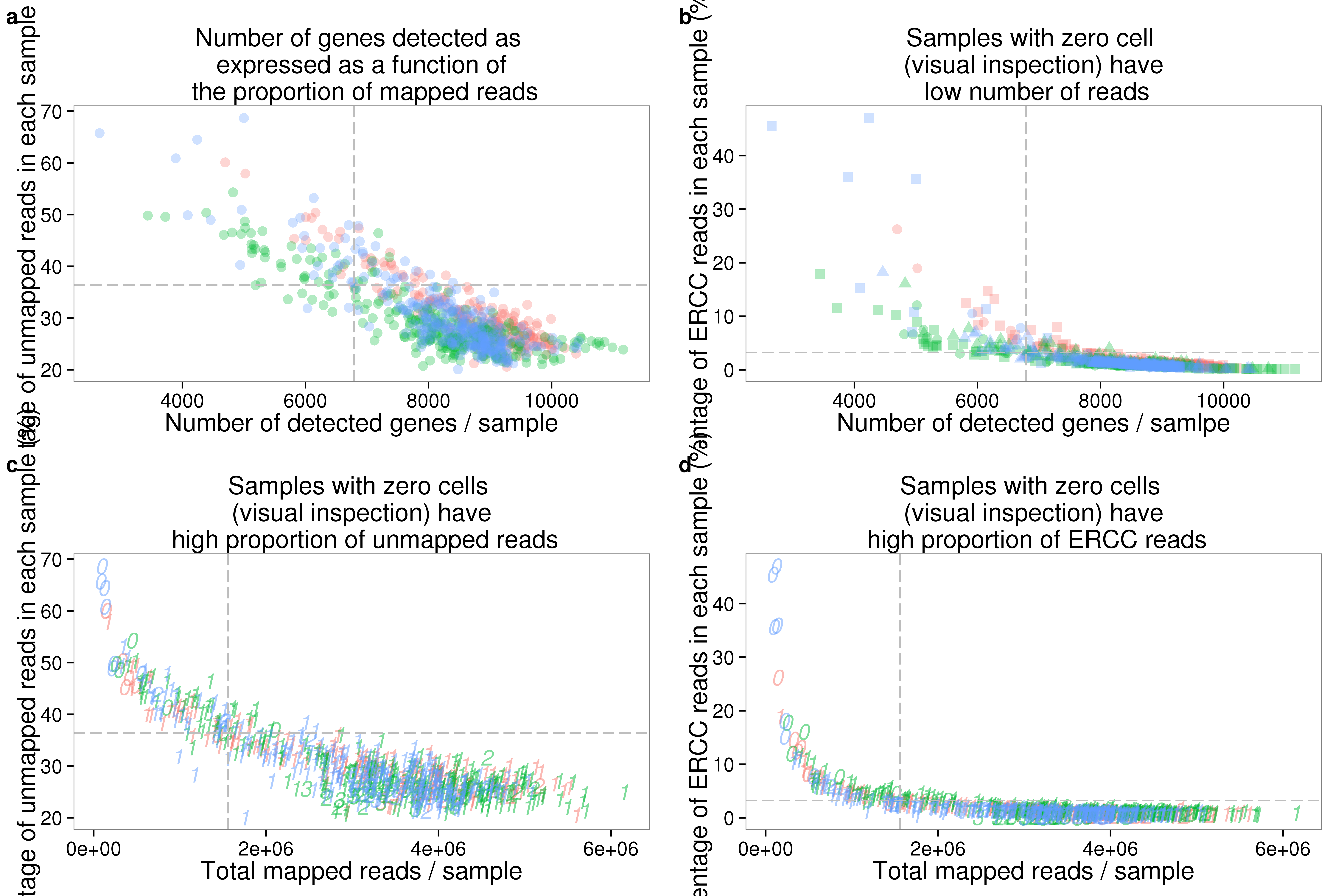plot_grid(genes_unmapped + theme(legend.position = c(.75,.9)) + labs(col = "Individual"),
labels = letters[3:5],
nrow = 1)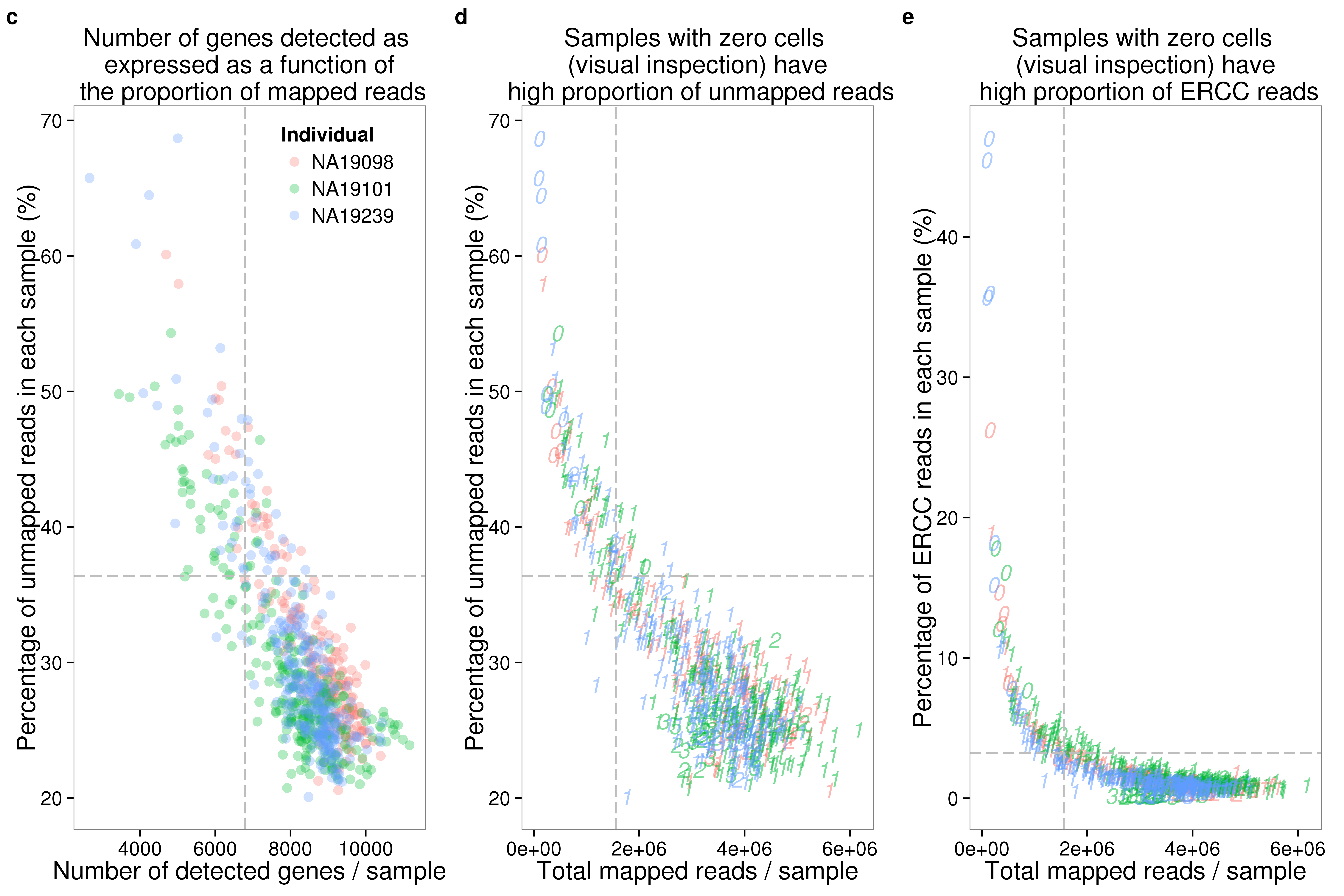plot_grid(ercc_reads_plot + theme(legend.position = "none"),
ercc_molecule_plot + theme(legend.position = "none"),
plot_unmapped + theme(legend.position = "none"),
plot_ercc + theme(legend.position = "none"),
plot_gene + theme(legend.position = "none"),
labels = letters[1:6],
ncol = 2)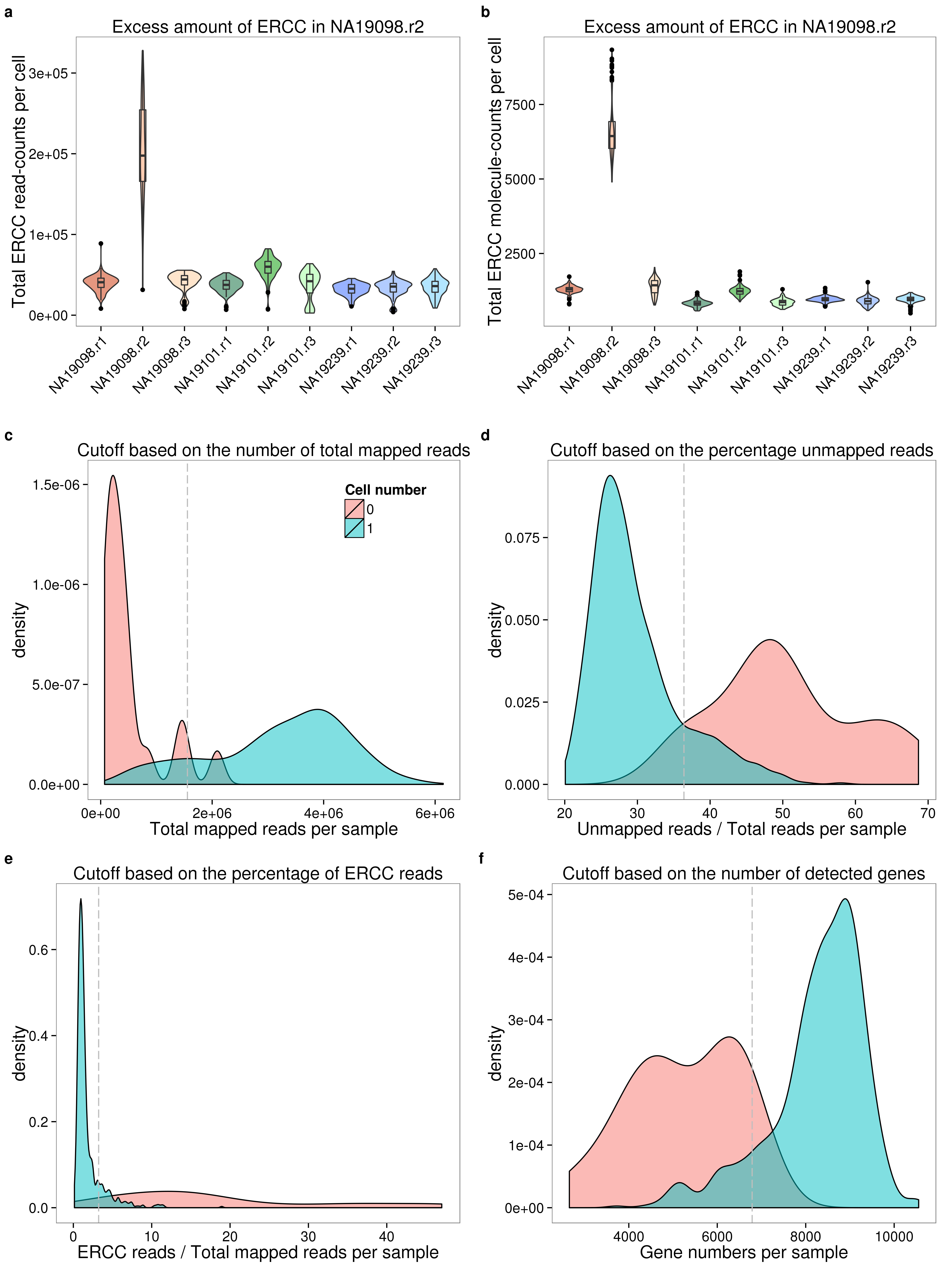plot_grid(plot_before + theme(legend.position=c(.85,.85)) + labs(col = "Cell number"),
plot_after + theme(legend.position = "none"),
plot_ercc_before,
plot_ercc_after,
labels = letters[1:4])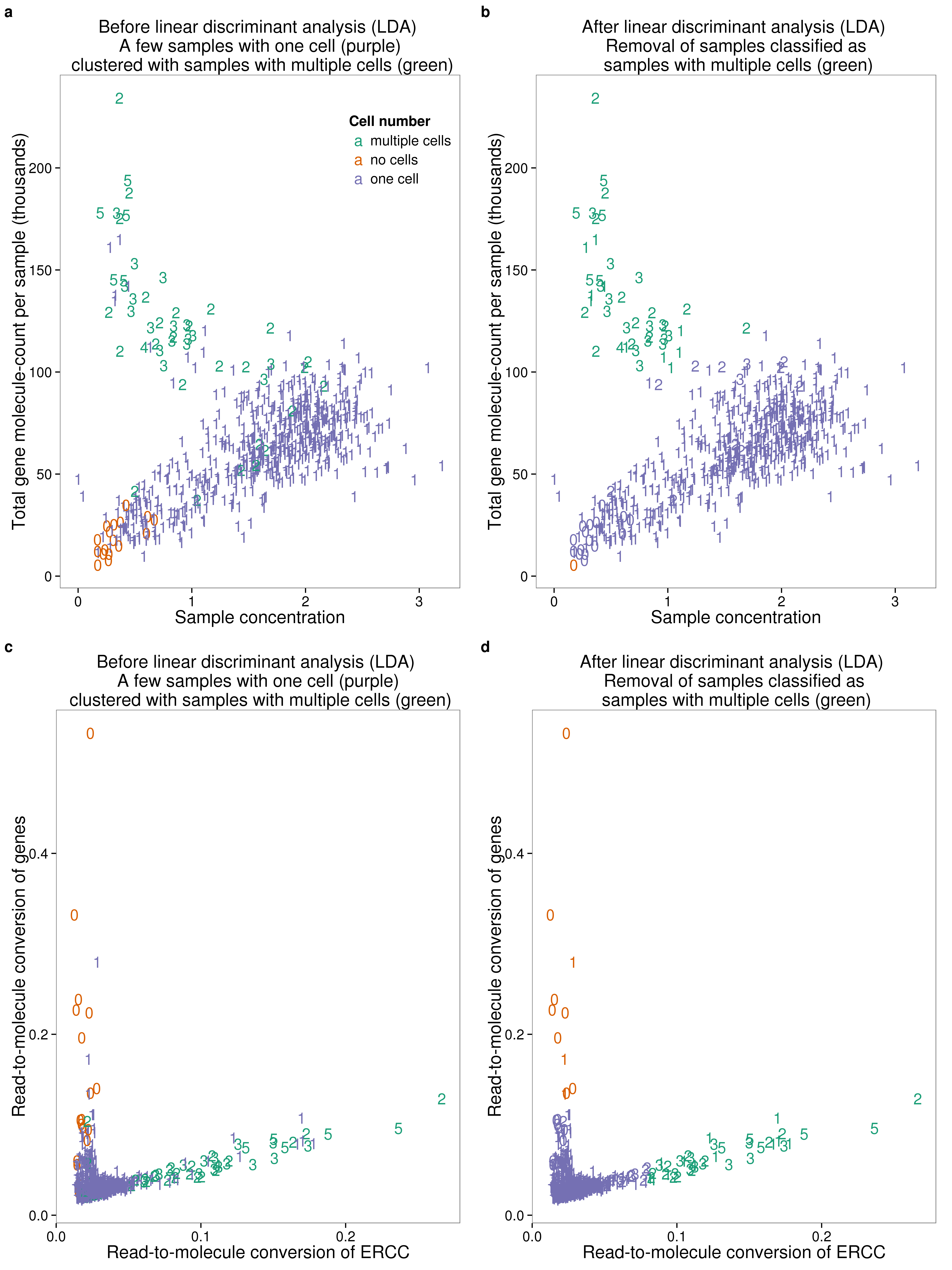## Session information

sessionInfo()
R version 3.2.0 (2015-04-16)
Platform: x86_64-unknown-linux-gnu (64-bit)

locale:
 LC_CTYPE=en_US.UTF-8       LC_NUMERIC=C
 LC_TIME=en_US.UTF-8        LC_COLLATE=en_US.UTF-8
 LC_MONETARY=en_US.UTF-8    LC_MESSAGES=en_US.UTF-8
 LC_PAPER=en_US.UTF-8       LC_NAME=C
 LC_MEASUREMENT=en_US.UTF-8 LC_IDENTIFICATION=C

attached base packages:
 stats     graphics  grDevices utils     datasets  methods   base

other attached packages:
 MASS_7.3-40   cowplot_0.3.1 ggplot2_1.0.1 edgeR_3.10.2  limma_3.24.9
 dplyr_0.4.2   knitr_1.10.5

loaded via a namespace (and not attached):
 Rcpp_0.12.4        magrittr_1.5       munsell_0.4.3
 colorspace_1.2-6   R6_2.1.1           stringr_1.0.0
 httr_0.6.1         plyr_1.8.3         tools_3.2.0
 parallel_3.2.0     grid_3.2.0         gtable_0.1.2
 DBI_0.3.1          htmltools_0.2.6    lazyeval_0.1.10
 yaml_2.1.13        assertthat_0.1     digest_0.6.8
 RColorBrewer_1.1-2 reshape2_1.4.1     formatR_1.2
 bitops_1.0-6       RCurl_1.95-4.6     evaluate_0.7
 rmarkdown_0.6.1    labeling_0.3       stringi_1.0-1
 scales_0.4.0       proto_0.3-10